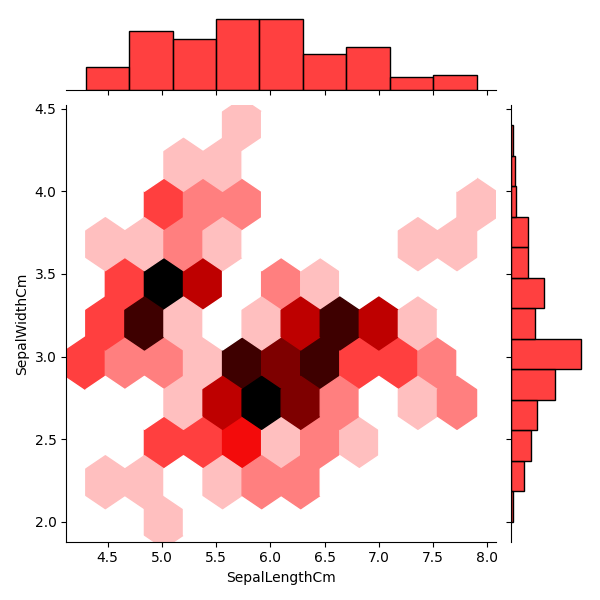﻿ Python Machine learning Scikit-learn: Create a joinplot to describe individual distributions on the same plot between Sepal length and Sepal width - w3resource# Python Scikit-learn: Create a joinplot to describe individual distributions on the same plot between Sepal length and Sepal width

## Python Machine learning Iris Visualization: Exercise-8 with Solution

Write a Python program to create a joinplot to describe individual distributions on the same plot between Sepal length and Sepal width.

Note: The bivariate analogue of a histogram is known as a “hexbin” plot, because it shows the counts of observations that fall within hexagonal bins. This plot works best with relatively large datasets. It’s available through the matplotlib plt.hexbin function and as a style in jointplot(). It looks best with a white background.

Sample Solution:

Python Code:

``````import pandas as pd
import seaborn as sns
import matplotlib.pyplot as plt
fig=sns.jointplot(x='SepalLengthCm', y='SepalWidthCm', kind="hex", color="red", data=iris)
plt.show()
```
```

Output:

``````

Python Code Editor:

Have another way to solve this solution? Contribute your code (and comments) through Disqus.

What is the difficulty level of this exercise?

﻿